﻿ GRACE-FO卫星星间指向定标与性能评估文章快速检索 高级检索
 大地测量与地球动力学2023, Vol. 43Issue (9): 881-886  DOI: 10.14075/j.jgg.2023.09.001### 引用本文HUANG Zhiyong, LI Shanshan, LI Shizhong, et al. Inter-Satellite Pointing Calibration and Performance Evaluation of GRACE-FO Satellite[J]. Journal of Geodesy and Geodynamics, 2023, 43(9): 881-886.### Foundation support

National Natural Science Foundation of China, No.42174007, 42204009.

### Corresponding author

LI Shanshan, professor, PhD supervisor, majors in physical geodesy, E-mail:zzy_lily@sina.com.

### 第一作者简介

HUANG Zhiyong, PhD candidate, majors in satellite gravity, E-mail:geo_hzy@126.com.

### 文章历史

GRACE-FO卫星星间指向定标与性能评估

1. 信息工程大学地理空间信息学院，郑州市科学大道62号，450001;
2. 地理信息工程国家重点实验室，西安市雁塔路中段1号，710054

GRACE型卫星双星Z轴不对地定向，而是偏离径向约1°，用以实现双星严格互瞄。由于其主载荷星间测距仪(K-band ranging assembly，KBR)相位中心、星敏感器安装矩阵在卫星入轨后发生变化，可能导致星间指向出现系统性偏差，该偏差可通过星间指向定标进行确定，并上注(GRACE卫星仅在2003年上注)至卫星姿轨控系统[1-2]，以实现准确的星间指向。星间指向性能评估可以监视星间指向状态，评估卫星姿控性能，辅助评估载荷状态，识别卫星机动事件。

Bandikova等的研究表明，星间指向角定标参数的确定有在轨和地面两条实现途径：对于星间指向定标来说，一般通过在轨途径; 对于星间指向监视与评估，一般通过地面途径。然而，其并未给出在轨路径的实现策略。

1 GRACE型卫星星间指向维持策略

GRACE-FO卫星姿轨控系统配备有12个10 mN的姿控推力器和2个50 mN的轨控推力器，同时，卫星每轴配备有磁偶极矩为27.5 mA2的磁力矩器作为主要的姿控器件。磁力矩器(magnetic torque，MTQ)三轴与卫星星体三轴平行安装，三轴磁力矩棒由圆柱形芯体和2个线圈组成。通过对指定轴向施加电流，能够产生该方向的磁力偶m，当与地球磁场B产生相互作用时，便能产生所需磁力矩T=m×B。为了实现双星互瞄，卫星需获得目标姿态，A星通过调整自身姿态至目标姿态，使得卫星KBR天线相位中心指向B星;B星以同样方式指向A星。由于AB星之间无数据链路，因此无法获得对方的位置，必须从地面上传AB星两行根数至双星，通过星上轨道预报计算对方星的位置，同时计算卫星视线方向(LOS，line-of-sight)连线作为目标姿态，通过姿轨控系统使得卫星姿态与视线方向一致。表 1 星间指向相关的1B级数据 Tab. 1 Level-1B data related to inter-satellite pointing
2 星间指向在轨定标及性能评估算法 2.1 星间指向角的含义

 $\boldsymbol{R}_{\mathrm{KF} \rightarrow \mathrm{LOS}}=\boldsymbol{R}_x(\psi) \boldsymbol{R}_y(\theta) \boldsymbol{R}_z(\varphi)$ (1)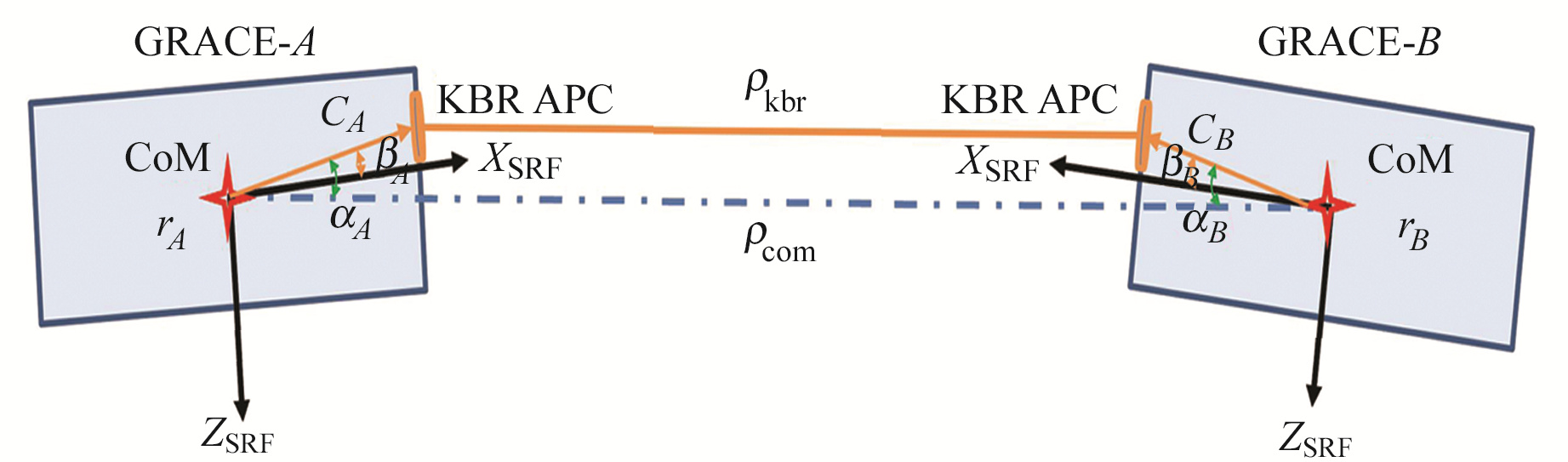图 1 GRACE型卫星星间指向角示意图 Fig. 1 Diagram of inter-satellite pointing angle of GRACE satellite

 \begin{aligned} & \boldsymbol{R}_{\mathrm{KF} \rightarrow \mathrm{LOS}}=\left[\begin{array}{c} \cos \theta \cos \varphi \\ -\cos \psi \sin \varphi+\sin \psi \sin \theta \cos \varphi \\ \sin \psi \sin \varphi+\cos \psi \sin \theta \cos \varphi \end{array}\right. \\ & \left.\begin{array}{cc} \cos \theta \sin \varphi & -\sin \theta \\ \cos \psi \cos \varphi+\sin \psi \sin \theta \sin \varphi & \sin \psi \cos \theta \\ -\sin \psi \cos \varphi+\cos \psi \sin \theta \sin \varphi & \cos \psi \cos \theta \end{array}\right] \\ & \end{aligned} (2)

 $\left\{\begin{array}{l} \psi=-\arctan \left(\frac{R_{23}}{R_{33}}\right) \\ \theta=\arcsin \left(R_{13}\right) \\ \varphi=-\arctan \left(\frac{R_{12}}{R_{11}}\right) \end{array}\right.$ (3)

2.2 在轨路径星间指向定标图 2 星间指向角计算的2种方法 Fig. 2 Two methods for calculating the inter-satellite pointing angle

 $\boldsymbol{R}_{\mathrm{KF} \rightarrow \mathrm{LOS}}=\boldsymbol{R}_{\text {iner } \rightarrow \mathrm{LOS}} \cdot\left(\boldsymbol{R}_{\text {iner } \rightarrow \mathrm{SCF}}\right)^{\mathrm{T}} \cdot\left(\boldsymbol{R}_{\mathrm{SCF} \rightarrow \mathrm{KF}}\right)^{\mathrm{T}}$ (4)

1) 通过式(4)计算自上次QKS1B更新以来的星间指向角方向余弦矩阵RKF→LOS，选取数据时排除质心定标机动、KBR定标机动等大的姿态机动等事件影响段全天的数据;

2) 通过式(3)计算对应的欧拉角，并计算欧拉角的均值ψ0θ0φ0;

3) 通过式(2)计算QKS1B的修正矩阵RΔQKS=Rx(－ψ0)Ry(－θ0)Rz(－φ0);

4) 计算新的QKS1B方向余弦矩阵RQKS1B=RSCF→KF·R TΔQKS1B;

4) 将RQKS1B转换为四元数形式，得到更新后的QKS1B，上注至卫星姿轨控分系统。

2.3 地面路径星间指向精度评估

 $\boldsymbol{R}_{\mathrm{KF} \rightarrow \mathrm{LOS}}=\boldsymbol{R}_{\text {iner } \rightarrow \mathrm{LOS}} \cdot\left(\boldsymbol{R}_{\text {iner } \rightarrow \mathrm{KF}}\right)^{\mathrm{T}}$ (5)

 $\boldsymbol{R}_{\text {iner } \rightarrow \mathrm{KF}}=\left[\begin{array}{c} \boldsymbol{R}_{\mathrm{SRF} \rightarrow \text { iner }} \frac{\mathrm{pc}}{|\mathrm{pc}|} \\ \boldsymbol{Z}_{\mathrm{KF}} \times \boldsymbol{X}_{\mathrm{KF}} \\ \boldsymbol{X}_{\mathrm{KF}} \times \boldsymbol{y}_{\mathrm{SRF}} \end{array}\right]$ (6)

 $\boldsymbol{R}_{\text {iner } \rightarrow \mathrm{LOS}}=\left[\begin{array}{c} \frac{r_i-r_j}{\left|r_i-r_j\right|} \\ x_{\mathrm{LOS}_j} \times \frac{r_A}{\left|r_A\right|} \\ x_{\mathrm{LOS}_j} \times y_{\mathrm{LOS}_j} \end{array}\right]$ (7)

3 GRACE-FO卫星星间指向性能评估

3.1 星间指向精度评估

 $\beta=\arccos \left(\frac{n \cdot r_{\odot}}{|n| \cdot r_{\odot}}\right)-90^{\circ}$ (8)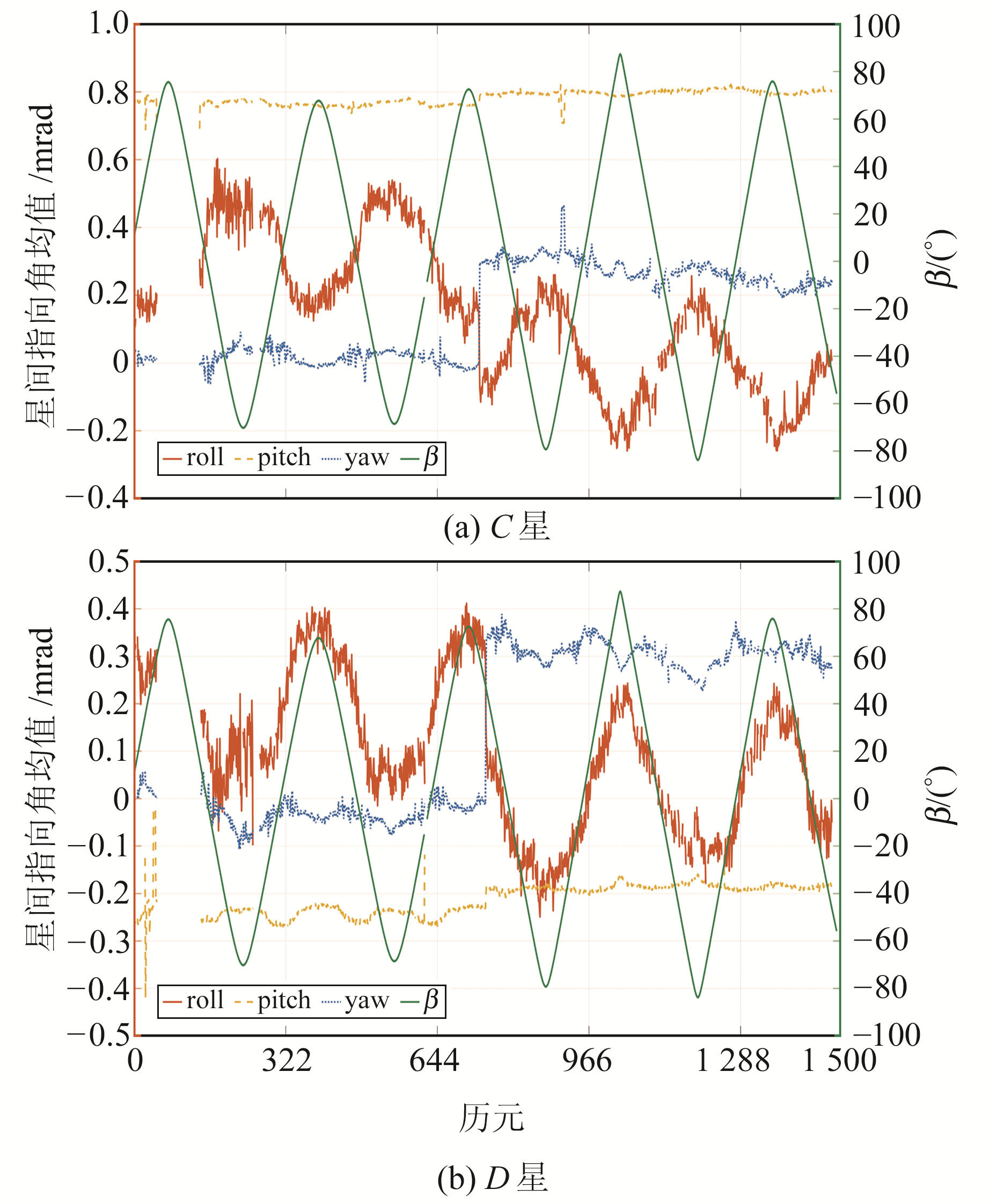图 3 GRACE-FO卫星2018-06-01~2022-06-23期间星间指向角均值 Fig. 3 The mean value of inter-satellite pointing angle of GRACE-FO from 2018-06-01 to 2022-06-23表 2 自发射以来的星间指向角标准差 Tab. 2 The standard deviation of the inter-satellite pointing since launch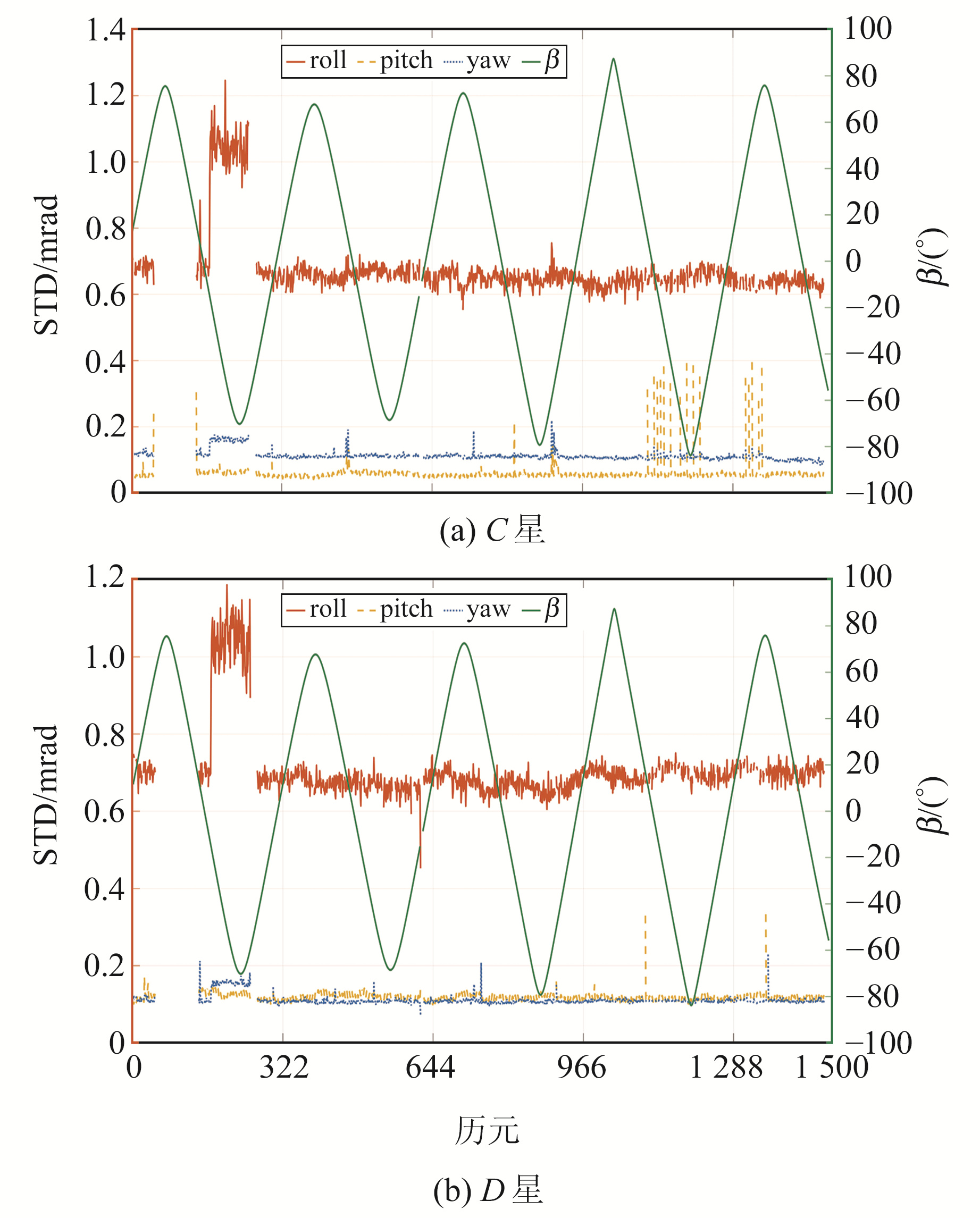图 4 GRACE-FO卫星2018-06-01~2022-06-23的星间指向角标准差 Fig. 4 The STD of inter-satellite pointing angle of GRACE-FO from 2018-06-01 to 2022-06-23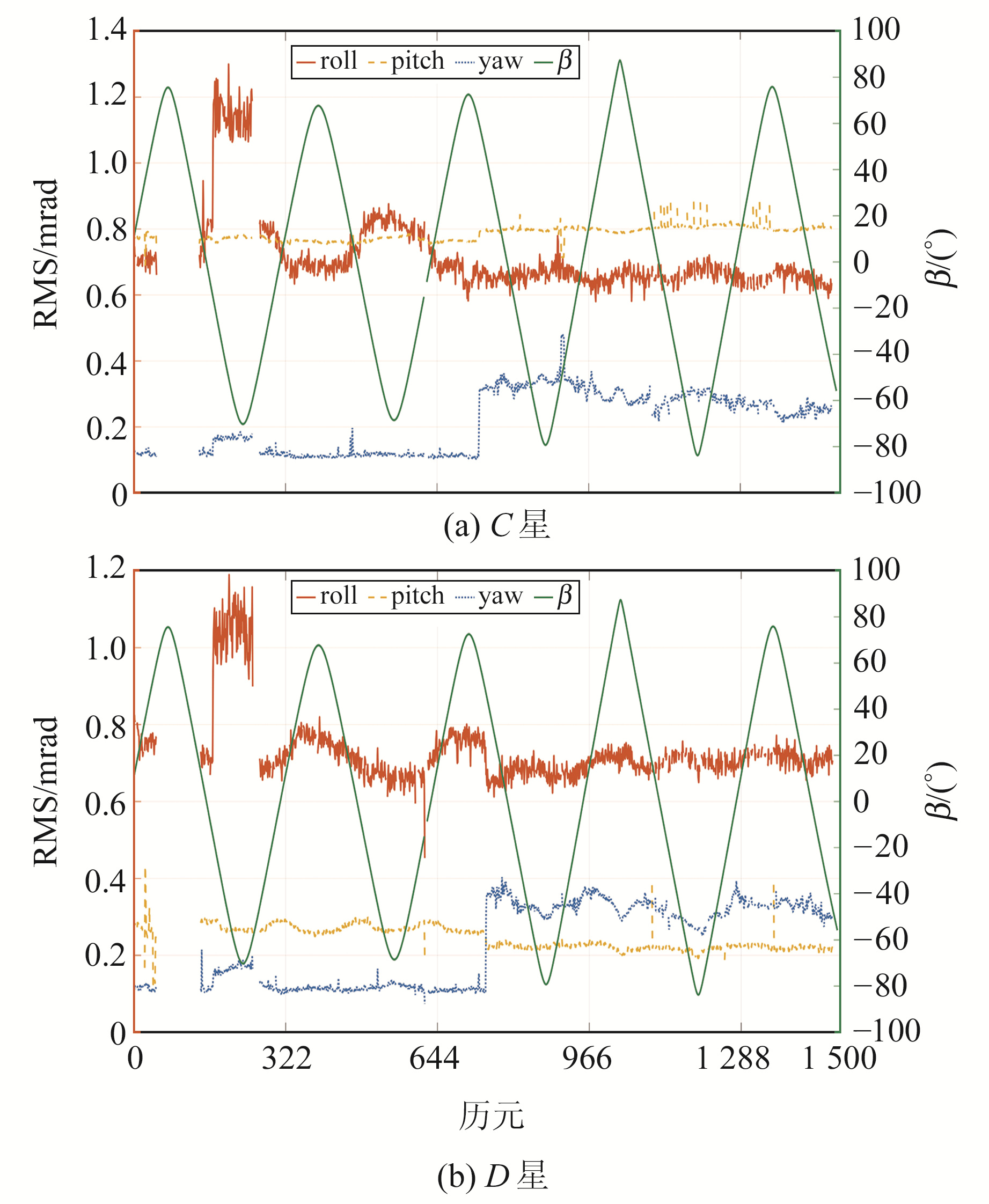图 5 GRACE-FO卫星2018-06-01~2022-06-23的星间指向角RMS Fig. 5 The RMS of inter-satellite pointing angle of GRACE-FO from 2018-06-01 to 2022-06-23
3.2 姿控能力评估图 6 C星2021-11-04~21的星间指向角RMS和磁场强度 Fig. 6 The RMS of inter-satellite pointing angle of GRACE-C from 2021-11-04 to 2021-11-21 and magnetic field strength表 3 磁力矩器在不同纬度的姿控能力 Tab. 3 The attitude control ability of magnetic torquer at different latitudes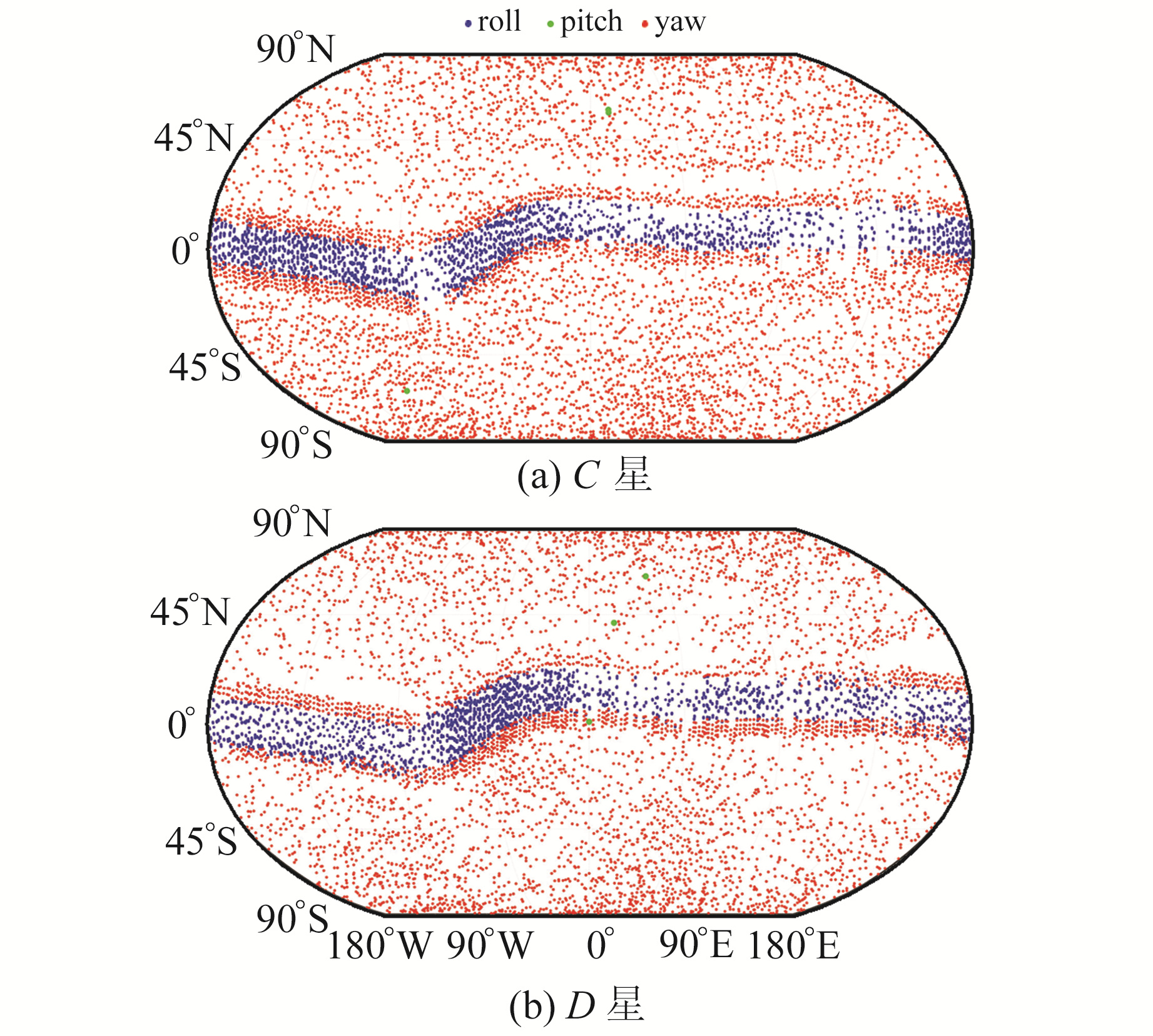图 7 GRACE-FO卫星2021-11-04~21的姿控点火事件 Fig. 7 The thruster events of GRACE-FO from 2021-11-04 to 2021-11-21
4 结语

1) 基于地面路径的星间指向评估能够识别星间指向角常偏，对星间指向定标有借鉴意义。

2) 2020-06-02C星的2、3星敏安装矩阵(QSA1B)更新后，星间指向角常偏略微变大。

3) roll方向星间指向角均值与β角有强相关性。

4) 从星间指向角统计的标准差和RMS可以看出，GRACE-FO卫星的星间指向满足要求，保证了KBR正常工作所需的基本条件。

5) GRACE-FO卫星姿轨控系统的磁力矩和姿控推力器能够配合完成姿控需求。

  Kornfeld, R P, Arnold, B W, Gross, M A, et al. GRACE-FO: The Gravity Recovery and Climate Experiment Follow-On Mission[J]. Journal of Spacecraft and Rockets, 2019, 56(3): 931-951 DOI:10.2514/1.A34326 (0)  Bandikova T. The Role of Attitude Determination for Inter-Satellite Ranging[D]. Hannover: Leibniz University of Hannover, 2015 (0)  Bandikova T, Flury J, Ko U D. Characteristics and Accuracies of the GRACE Inter-Satellite Pointing[J]. Advances in Space Research, 2012, 50(1): 123-135 DOI:10.1016/j.asr.2012.03.011 (0)  闫易浩. GRACE/GRACE-FO重力卫星星间测距系统数据处理关键技术研究[D]. 武汉: 华中科技大学, 2021 (Yan Yihao. Research on the Raw Data Processing Technology of the Inter-Satellite Ranging System of GRACE/GRACE-FO Gravimetric Missions[D]. Whuhan: Huazhong University of Science and Technology, 2021)) (0)  Goswami S, Francis S P, Bandikova T, et al. Analysis of GRACE Follow-On Laser Ranging Interferometer Derived Inter-Satellite Pointing Angles[J]. IEEE Sensors Journal, 2021, 21(17): 19 209-19 221 DOI:10.1109/JSEN.2021.3090790 (0)  Schlepp B, Kirschner M, Sweetser T H, et al. Flight Dynamics Challenges for the GRACE Follow-On Mission[C]. 25th International Symposium on Space Flight Dynamics(ISSFD), Munich, 2015 (0)  Wen H Y, Kruizinga G, Paik M, et al. Gravity Recovery and Climate Experiment Follow-On(GRACE-FO) Level-1 Data Product User Handbook; Technical Report JPL, D-56935[R]. NASA Jet Propulsion Laboratory/California Institute of Technology: Pasadena, 2019 (0)  Cossavella F, Herman J, Hoffmann L, et al. Attitude Control on GRACE Follow-On: Experiences from the First Years in Orbit[M]. Cham: Springer, 2022 (0)  Huang Z Y, Li S S, Cai L, et al. Estimation of the Center of Mass of GRACE-Type Gravity Satellites[J]. Remote Sensing, 2022(14): 4 030 (0)  Huang Z Y, Li S S, Huang L Y, et al. On-Orbit Calibration of the KBR Antenna Phase Center of GRACE-Type Gravity Satellites[J]. Remote Sensing, 2022(14): 3 395 (0)
Inter-Satellite Pointing Calibration and Performance Evaluation of GRACE-FO Satellite
HUANG Zhiyong1,2LI Shanshan1LI Shizhong2     HUANG Lingyong2     ZHU Leiming2     TAN Xuli1     PAN Xingchen2     NIU Hanhan2
1. School of Surveying and Mapping, Information Engineering University, 62 Kexue Road, Zhengzhou 450001, China;
2. State Key Laboratory of Geo-Information Engineering, 1 Mid-Yanta Road, Xi 'an 710054, China
Abstract: Since the KBR phase center and the installation matrix of the star sensor may change after the satellites enter the orbit, there will be a systematic bias of the inter-satellite pointing of GRACE-FO satellite. In order to correct the bias, it is necessary to uplink the inter-satellite pointing calibration parameters into the satellite attitude and orbit control system. In this paper, we derive the correlative algorithms of inter-satellite pointing calibration and accuracy evaluation, and propose the process and implementation strategy of inter-satellite pointing calibration based on in-flight path. Based on the post-processing path, we evaluate the inter-satellite pointing performance of GRACE-FO since its launch. The STD and RMS of the inter-satellite pointing angle indicate that the GRACE-FO inter-satellite pointing meets the requirements of pitch and yaw direction 1 mrad and roll direction 10 mrad for the normal operation of KBR. The magnetic torque of the satellite attitude and orbit control system and the attitude control thruster can meet the requirements of attitude control.
Key words: inter-satellite pointing; GRACE; GRACE-FO; calibration; attitude control